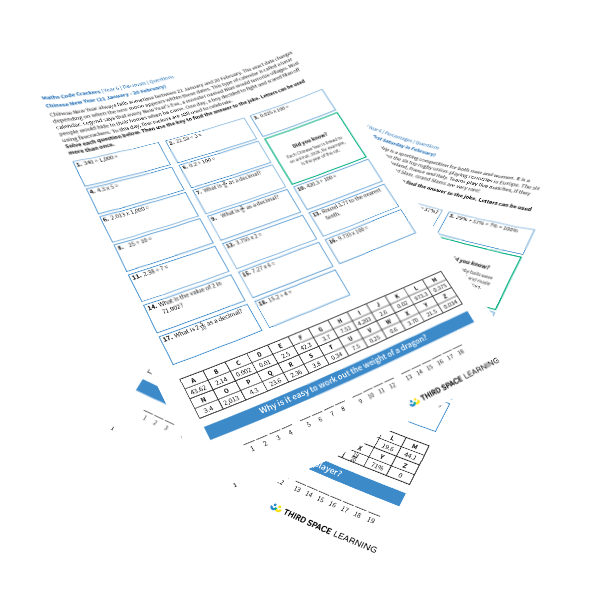# Maths Code Crackers Year 6 Spring Pack

Recap knowledge with an engaging end of block retrieval activity where pupils crack a code to find the punchline to a joke.

A set of six worksheets designed to be used at the end of each White Rose block in Spring term. The activities are based around retrieval of knowledge. Pupils will solve a series of questions to find the punchline to a joke related to a day in the Spring term.

Pack contains:

• One decimals worksheet
• One percentages worksheet
• One algebra worksheet
• One converting units worksheet
• One perimeter, area and volume worksheet
• One ratio worksheet

Year 6

Decimals, Percentages, Algebra, Measurement, Ratio and Proportion